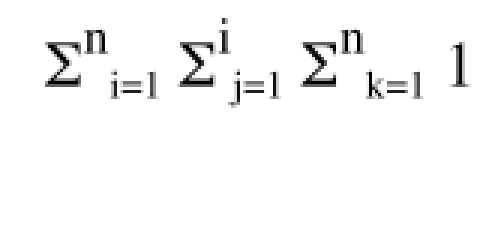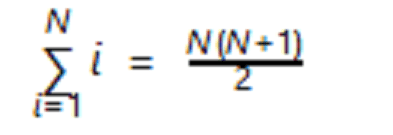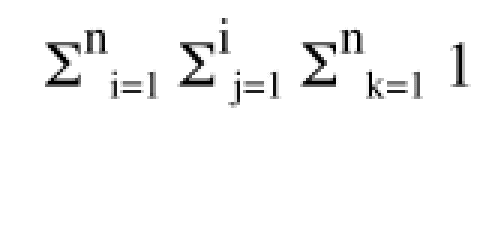# Summation problem (first N positive integers)

## Homework Statement## Homework Equations

so i kno the formula for the for the sum of the first N positive integerswhen i = 1

## The Attempt at a Solution

i kno the answer = n^2(n+1)/2

but could someone explain step by step how you reduce it to get the final answer? as if i'm in kindergarten? i'm slow, thanks.

my work:

following the formula: n(n+1)/2 * n(n+1)/2 * n(n+1)/2 ?

i don't understand how to reduce the terms to get the final answer provided

Last edited:

Ray Vickson
Homework Helper
Dearly Missed

## Homework Statement## Homework Equations

so i kno the formula for the for the sum of the first N positive integerswhen i = 1

## The Attempt at a Solution

i kno the answer = n^2(n+1)/2

but could someone explain step by step how you reduce it to get the final answer? as if i'm in kindergarten? i'm slow, thanks.

PF rules require you to show us your work.

oh okay sure

rcgldr
Homework Helper
Are you sure the upper bound on the second summation is "i" and not "n"? Note that this is a summation of the value "1", not the variable "i".

Dick